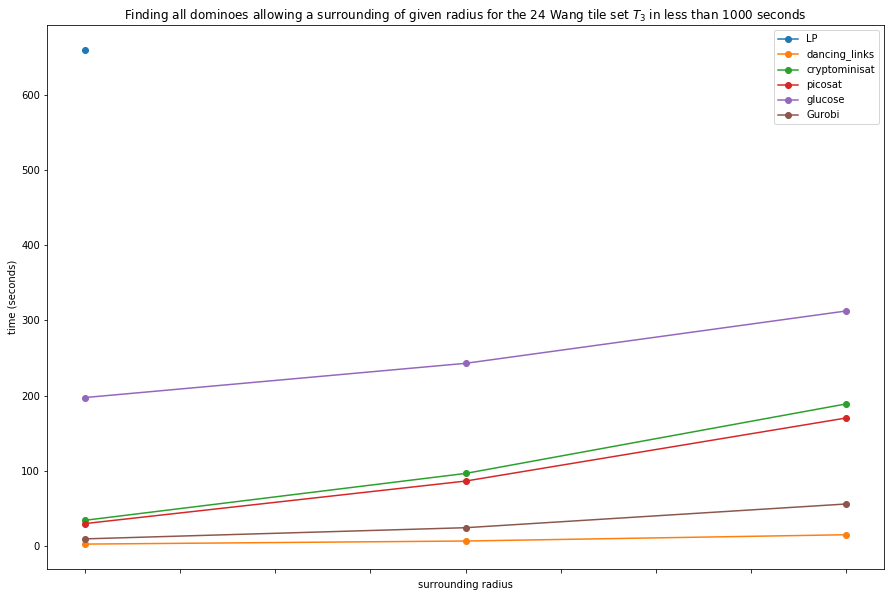In :
%matplotlib inline


# Dependencies¶

This notebook depends on the most recent development version of (slabbe)[https://pypi.python.org/pypi/slabbe] Optional Sage Package. The latest release can be installed with sage -pip install slabbe.

This notebook depends on the use of Glucose as a Sage optional package: https://trac.sagemath.org/ticket/26361

In :
from slabbe import WangTileSet


# Jeandel-Rao's 11 Wang tiles¶

We construct Jeandel-Rao's set of Wang tiles $T_0$ as well at tile sets $T_2$ and $T_3$ from https://arxiv.org/abs/1808.07768

In :
tiles = [(2,4,2,1), (2,2,2,0), (1,1,3,1), (1,2,3,2), (3,1,3,3),
(0,1,3,1), (0,0,0,1), (3,1,0,2), (0,2,1,2), (1,2,1,4), (3,3,1,2)]
T0 = WangTileSet([map(str,t) for t in tiles])
T0.tikz()

Out: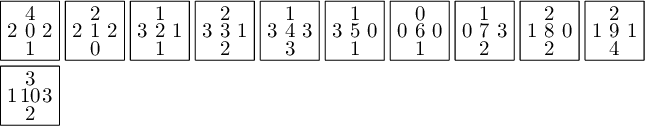In :
tiles = [(0, 0, 0, 1), (0, 1, 3, 1), (0, 2, 1, 2), (1, 1, 3, 1), (1, 2, 3, 2), (3, 1, 0, 2), (3, 1, 3, 3), (3, 3, 1, 2),
(203, 1, 210, 0), (203, 3, 211, 0), (210, 2, 211, 1), (210, 2, 231, 0), (211, 2, 213, 1),
(211, 2, 233, 0), (213, 1, 210, 1), (213, 1, 230, 0), (213, 3, 211, 1), (230, 1, 203, 0),
(231, 1, 203, 0), (233, 1, 213, 0)]
T2 = WangTileSet([map(str,t) for t in tiles])
T2.tikz()

Out: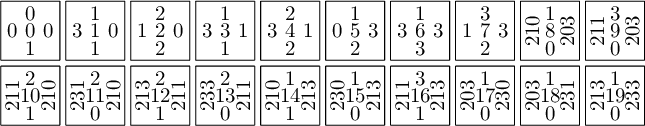In :
tiles = [(0, 0, 0, 1), (0, 1, 3, 1), (3, 1, 0, 2), (3, 1, 3, 3), (2030, 1, 2103, 0), (2033, 1, 2113, 0),
(2103, 1, 2110, 1), (2103, 1, 2310, 0), (2103, 3, 2111, 1), (2110, 2, 2131, 1), (2110, 2, 2331, 0), (2111, 2, 2133, 1),
(2113, 1, 2130, 1), (2113, 1, 2330, 0), (2130, 0, 2300, 0), (2130, 1, 2103, 1), (2131, 1, 2103, 1), (2133, 1, 2113, 1),
(2300, 0, 2030, 0), (2300, 1, 2033, 0), (2310, 1, 2033, 0), (2330, 0, 2130, 0), (2330, 1, 2133, 0), (2331, 1, 2133, 0)]
T3 = WangTileSet([map(str,t) for t in tiles])
T3.tikz()

Out: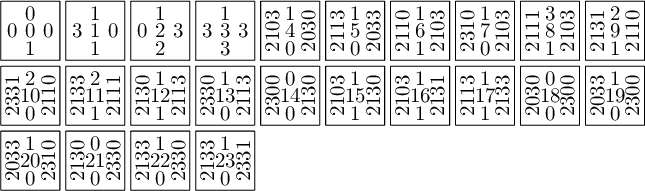# Tiling a 3x3 square with Wang tiles¶

In :
tiling = T0.solver(3,3).solve(solver='Coin')
tiling.tikz()

Out: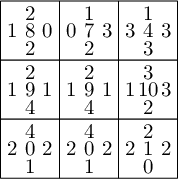In :
tiling = T0.solver(3,3).solve(solver='glucose')
tiling.tikz()

Out: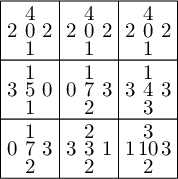## Reduction to SAT:¶

The reduction to SAT of the tiling of a $n\times n$ square by $t$ Wang tiles needs $t\times n^2$ variables and at most $2n^2 t^2$ constraints.

In :
for k in range(10,70,10):
print k, T0.solver(k,k).sat_solver('cryptominisat')

10 CryptoMiniSat solver: 1100 variables, 21440 clauses.
20 CryptoMiniSat solver: 4400 variables, 89280 clauses.
30 CryptoMiniSat solver: 9900 variables, 203520 clauses.
40 CryptoMiniSat solver: 17600 variables, 364160 clauses.
50 CryptoMiniSat solver: 27500 variables, 571200 clauses.
60 CryptoMiniSat solver: 39600 variables, 824640 clauses.


## Reduction to MILP¶

The reduction to MILP of the tiling of a $n\times n$ square needs $11\times n^2$ variables and $2n(n-1)+n^2$ constraints.

In :
for k in range(10,70,10):
print k, T0.solver(k,k).milp()

10 (Boolean Program (maximization, 1100 variables, 280 constraints), MIPVariable of dimension 1)
20 (Boolean Program (maximization, 4400 variables, 1160 constraints), MIPVariable of dimension 1)
30 (Boolean Program (maximization, 9900 variables, 2640 constraints), MIPVariable of dimension 1)
40 (Boolean Program (maximization, 17600 variables, 4720 constraints), MIPVariable of dimension 1)
50 (Boolean Program (maximization, 27500 variables, 7400 constraints), MIPVariable of dimension 1)
60 (Boolean Program (maximization, 39600 variables, 10680 constraints), MIPVariable of dimension 1)


# Finding a nxn valid Wang square tiling : comparing solvers¶

In :
import time,signal
def time_solving_squares(wang_tile_set, solver='gurobi', maxsize=1000, step=1, timelimit=5, verbose=False):
r"""
Return a list of tuples (n,t) where t is the time spent (in seconds) finding
a valid Wang tiling of the n times n square.

EXAMPLES::

sage: time_solving_squares(solver='gurobi', timelimit=1)
[(1, 0.0019371509552001953),
(2, 0.004050016403198242),
(3, 0.01121377944946289),
(4, 0.015249013900756836),
(5, 0.0812530517578125),
(6, 0.08473992347717285),
(7, 0.23214101791381836),
(8, 0.33846092224121094),
(9, 0.6193099021911621),
(10, 1)]
"""
L = []
for i in range(1, maxsize, step):
try:
signal.alarm(timelimit)
start = time.time()
solution = wang_tile_set.solver(i,i).solve(solver=solver, ncpus=8)
end = time.time()
except (AlarmInterrupt, ValueError): # dancing_links catches the alarm and returns valueError
L.append( (i,timelimit) )
break
else:
signal.alarm(0)    # cancels the alarm
duration = end - start
L.append( (i,duration) )
if verbose:
print( (i,duration) )
return L


Testing:

In :
time_solving_squares(T0, solver='Gurobi', timelimit=1)

Out:
[(1, 0.0034780502319335938),
(2, 0.00877690315246582),
(3, 0.013873100280761719),
(4, 0.020534038543701172),
(5, 0.16086292266845703),
(6, 0.08809590339660645),
(7, 0.2522618770599365),
(8, 0.38977479934692383),
(9, 0.7161390781402588),
(10, 1)]
In :
import pandas as pd
def compare_solvers(wang_tile_set, solvers, maxsize=1000, step=1, timelimit=5):
wang_tile_set = WangTileSet(list(wang_tile_set)) # reinitialize cached values
series_dict = {}
for solver in solvers:
print('Testing solver {} ...'.format(solver))
L = time_solving_squares(wang_tile_set, solver=solver, maxsize=maxsize, step=step, timelimit=timelimit)
index,data = zip(*L)
series = pd.Series(data=data, index=index, dtype=float)
series_dict[solver] = series
print('    -> solved {} instances'.format(len(series)))
sorted_solvers = sorted(solvers, key=lambda solver:len(series_dict[solver]))
return pd.DataFrame(series_dict, columns=sorted_solvers)


Define the list of solvers:

In :
LPsolvers = ['Gurobi', 'LP']
slowLPsolvers = ['GLPK', 'PPL']
SATsolvers = ['cryptominisat', 'picosat', 'glucose']
uninstalled = ['Coin', 'GLPK/exact', 'CPLEX', 'CVXOPT', 'rsat']  # to be added to the comparison one day
solvers = slowLPsolvers + SATsolvers + LPsolvers + ['dancing_links']

In :
df_square_T0 = compare_solvers(T0, solvers, timelimit=10)

Testing solver GLPK ...
-> solved 7 instances
Testing solver PPL ...
-> solved 5 instances
Testing solver cryptominisat ...
-> solved 42 instances
Testing solver picosat ...
-> solved 37 instances
Testing solver glucose ...
-> solved 56 instances
Testing solver Gurobi ...
-> solved 13 instances
Testing solver LP ...
-> solved 15 instances
-> solved 23 instances

In :
ax = df_square_T0.plot(figsize=(15,10), marker='o')
ax.set(xlabel="n, the square size", ylabel="time (seconds)",
title='Time to find a valid Wang Tiling of a n x n square using Jeandel Rao 11 tile set $T_0$')
ax.get_figure().savefig('T0_square_tilings.svg')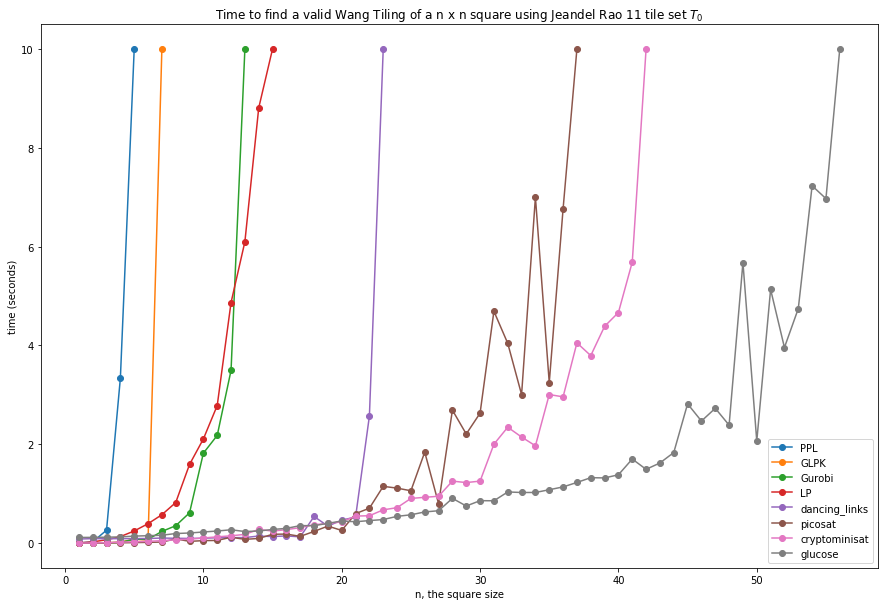In :
df_square_T2 = compare_solvers(T2, solvers, timelimit=10)

Testing solver GLPK ...
-> solved 6 instances
Testing solver PPL ...
-> solved 4 instances
Testing solver cryptominisat ...
-> solved 35 instances
Testing solver picosat ...
-> solved 24 instances
Testing solver glucose ...
-> solved 39 instances
Testing solver Gurobi ...
-> solved 11 instances
Testing solver LP ...
-> solved 11 instances
-> solved 22 instances

In :
ax = df_square_T2.plot(figsize=(15,10), marker='o')
ax.set(xlabel="n, the square size", ylabel="time (seconds)",
title='Time to find a valid Wang Tiling of a n x n square using the 20 Wang tile set $T_2$')
ax.get_figure().savefig('T2_square_tilings.svg')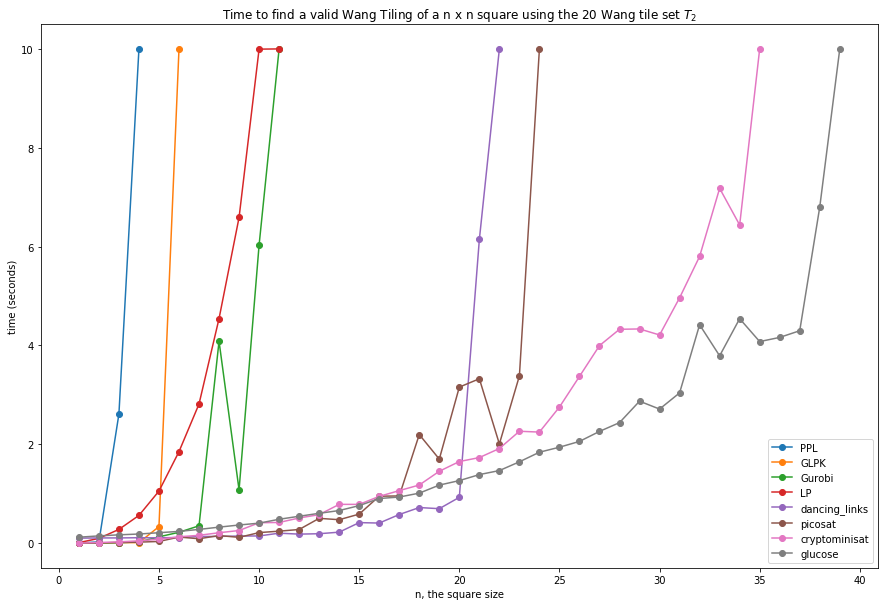In :
df_square_T3 = compare_solvers(T3, solvers, timelimit=10)

Testing solver GLPK ...
-> solved 6 instances
Testing solver PPL ...
-> solved 4 instances
Testing solver cryptominisat ...
-> solved 24 instances
Testing solver picosat ...
-> solved 27 instances
Testing solver glucose ...
-> solved 35 instances
Testing solver Gurobi ...
-> solved 11 instances
Testing solver LP ...
-> solved 9 instances
-> solved 16 instances

In :
ax = df_square_T3.plot(figsize=(15,10), marker='o')
ax.set(xlabel="n, the square size", ylabel="time (seconds)",
title='Time to find a valid Wang Tiling of a n x n square using the 24 Wang tile set $T_3$')
ax.get_figure().savefig('T3_square_tilings.svg')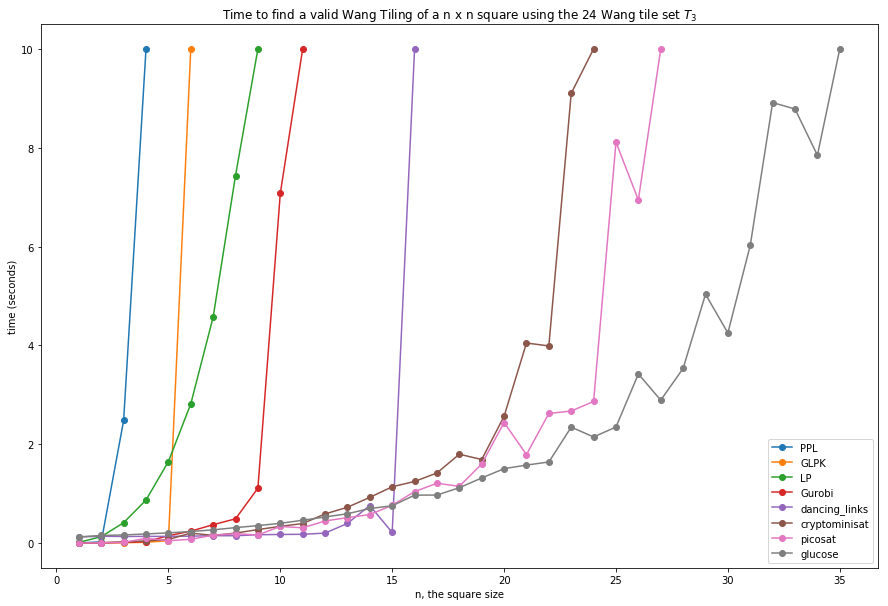# Finding all dominoes admitting a surrounding : comparing solvers¶

In :
import time,signal
def time_finding_dominoes(wang_tile_set, solver='gurobi', radius_list=[1,2,3], timelimit=5, verbose=False):
r"""
EXAMPLES::

sage: time_finding_dominoes(solver='gurobi', timelimit=30)
[(1, 2.8133392333984375e-05),
(2, 1.2874603271484375e-05),
(3, 5.506983995437622),
(4, 10.366616010665894),
(5, 20.101346015930176),
(6, 30)]
"""
L = []
try:
signal.alarm(timelimit)
start = time.time()
dominoes = {}
for direction in [1, 2]:
if verbose:
print('{} computes {} dominoes in the direction e{} with'
end = time.time()
except (AlarmInterrupt, ValueError): # dancing_links catches the alarm and returns valueError
break
else:
signal.alarm(0)    # cancels the alarm
duration = end - start
if verbose:
return L

In :
time_finding_dominoes(T2, solver='dancing_links', verbose=True, timelimit=10)

dancing_links computes 38 dominoes in the direction e1 with surrounding radius 1
dancing_links computes 64 dominoes in the direction e2 with surrounding radius 1
Computation time for radius 1: 1.491492033 seconds
dancing_links computes 35 dominoes in the direction e1 with surrounding radius 2
dancing_links computes 50 dominoes in the direction e2 with surrounding radius 2
Computation time for radius 2: 4.06505918503 seconds
dancing_links computes 35 dominoes in the direction e1 with surrounding radius 3

Out:
[(1, 1.4914920330047607, 38, 64), (2, 4.065059185028076, 35, 50)]
In :
time_finding_dominoes(T2, solver='picosat', verbose=True, timelimit=100)

picosat computes 38 dominoes in the direction e1 with surrounding radius 1
picosat computes 64 dominoes in the direction e2 with surrounding radius 1
Computation time for radius 1: 14.2032501698 seconds
picosat computes 35 dominoes in the direction e1 with surrounding radius 2
picosat computes 50 dominoes in the direction e2 with surrounding radius 2
Computation time for radius 2: 41.7534508705 seconds
picosat computes 35 dominoes in the direction e1 with surrounding radius 3
picosat computes 50 dominoes in the direction e2 with surrounding radius 3
Computation time for radius 3: 82.6413869858 seconds

Out:
[(1, 14.203250169754028, 38, 64),
(2, 41.753450870513916, 35, 50),
(3, 82.64138698577881, 35, 50)]
In :
import pandas as pd
def compare_solvers_for_dominoes(wang_tile_set, solvers, radius_list=[1,2,3], timelimit=5, verbose=False):
wang_tile_set = WangTileSet(list(wang_tile_set)) # reinitialize cached values
series_dict = {}
for solver in solvers:
print('Testing solver {} ...'.format(solver))
timelimit=timelimit, verbose=verbose)
if L:
index,data,dir1,dir2 = zip(*L)
print('    -> Found {} and {} dominoes in the direction'
print('    -> time data: {}'.format(data))
else:
index = []
data = []
series = pd.Series(data=data, index=index, dtype=float)
series_dict[solver] = series
sorted_solvers = sorted(solvers, key=lambda solver:len(series_dict[solver]))
return pd.DataFrame(series_dict, columns=sorted_solvers)

In :
solvers = ['dancing_links'] + SATsolvers + LPsolvers # + slowLPsolvers
timelimit = 1000

In :
df_dominoes_T0 = compare_solvers_for_dominoes(T0, solvers, radius_list=[1,2,3], timelimit=timelimit)
df_dominoes_T0

Testing solver dancing_links ...
-> Found (27, 27, 27) and (30, 29, 29) dominoes in the direction 1 and 2 resp for radius in [1, 2, 3]
-> time data: (0.2731480598449707, 0.6504981517791748, 1.2540009021759033)
Testing solver cryptominisat ...
-> Found (27, 27, 27) and (30, 29, 29) dominoes in the direction 1 and 2 resp for radius in [1, 2, 3]
-> time data: (1.4911208152770996, 4.444904804229736, 9.103967905044556)
Testing solver picosat ...
-> Found (27, 27, 27) and (30, 29, 29) dominoes in the direction 1 and 2 resp for radius in [1, 2, 3]
-> time data: (1.0466749668121338, 3.2454769611358643, 6.970124959945679)
Testing solver glucose ...
-> Found (27, 27, 27) and (30, 29, 29) dominoes in the direction 1 and 2 resp for radius in [1, 2, 3]
-> time data: (36.92062783241272, 38.37336015701294, 42.61258316040039)
Testing solver Gurobi ...
-> Found (27, 27, 27) and (30, 29, 29) dominoes in the direction 1 and 2 resp for radius in [1, 2, 3]
-> time data: (1.290088176727295, 2.8659470081329346, 5.688135862350464)
Testing solver LP ...
-> Found (27, 27, 27) and (30, 29, 29) dominoes in the direction 1 and 2 resp for radius in [1, 2, 3]
-> time data: (24.0501070022583, 71.82991909980774, 159.4241440296173)

Out:
dancing_links cryptominisat picosat glucose Gurobi LP
1 0.273148 1.491121 1.046675 36.920628 1.290088 24.050107
2 0.650498 4.444905 3.245477 38.373360 2.865947 71.829919
3 1.254001 9.103968 6.970125 42.612583 5.688136 159.424144
In :
ax = df_dominoes_T0.plot(figsize=(15,10), marker='o')
title='Finding the dominoes allowing a surrounding of given radius '
'for the Jeandel Rao 11 tile set $T_0$ in less than {} seconds'.format(timelimit))
ax.get_figure().savefig('T0_dominoes_surrounding.svg')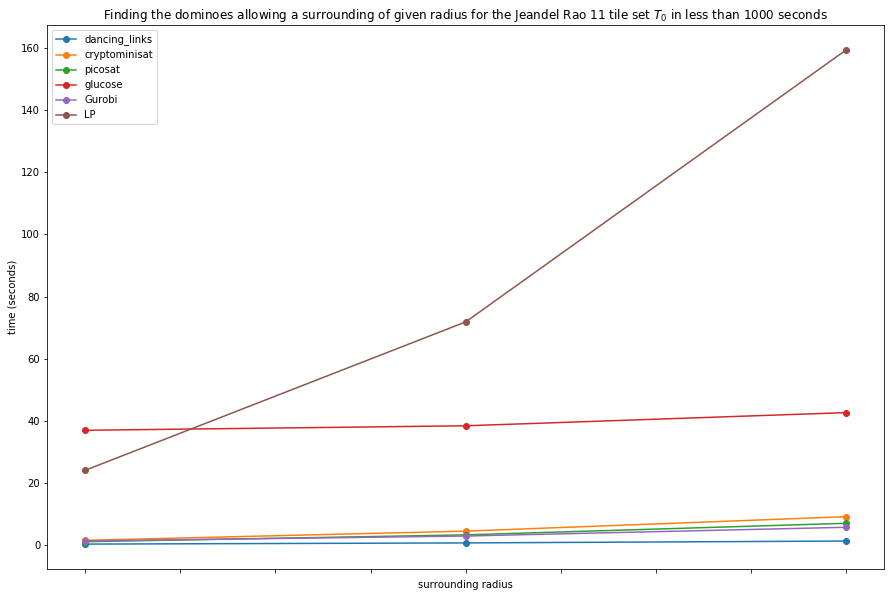In :
df_dominoes_T2 = compare_solvers_for_dominoes(T2, solvers, radius_list=[1,2,3], timelimit=timelimit)
df_dominoes_T2

Testing solver dancing_links ...
-> Found (38, 35, 35) and (64, 50, 50) dominoes in the direction 1 and 2 resp for radius in [1, 2, 3]
-> time data: (1.4719769954681396, 3.9624440670013428, 10.121387004852295)
Testing solver cryptominisat ...
-> Found (38, 35, 35) and (64, 50, 50) dominoes in the direction 1 and 2 resp for radius in [1, 2, 3]
-> time data: (17.726919889450073, 50.687167167663574, 101.87277793884277)
Testing solver picosat ...
-> Found (38, 35, 35) and (64, 50, 50) dominoes in the direction 1 and 2 resp for radius in [1, 2, 3]
-> time data: (15.524392127990723, 44.47639989852905, 86.34836101531982)
Testing solver glucose ...
-> Found (38, 35, 35) and (64, 50, 50) dominoes in the direction 1 and 2 resp for radius in [1, 2, 3]
-> time data: (128.16299200057983, 153.79267597198486, 190.46218085289001)
Testing solver Gurobi ...
-> Found (38, 35, 35) and (64, 50, 50) dominoes in the direction 1 and 2 resp for radius in [1, 2, 3]
-> time data: (5.814908027648926, 13.985903978347778, 30.073441982269287)
Testing solver LP ...
-> Found (38,) and (64,) dominoes in the direction 1 and 2 resp for radius in [1, 2, 3]
-> time data: (309.00411796569824,)

Out:
LP dancing_links cryptominisat picosat glucose Gurobi
1 309.004118 1.471977 17.726920 15.524392 128.162992 5.814908
2 NaN 3.962444 50.687167 44.476400 153.792676 13.985904
3 NaN 10.121387 101.872778 86.348361 190.462181 30.073442
In :
ax = df_dominoes_T2.plot(figsize=(15,10), marker='o')
title='Finding all dominoes allowing a surrounding of given radius '
'for the 20 Wang tile set $T_2$ in less than {} seconds'.format(timelimit))
ax.get_figure().savefig('T2_dominoes_surrounding.svg')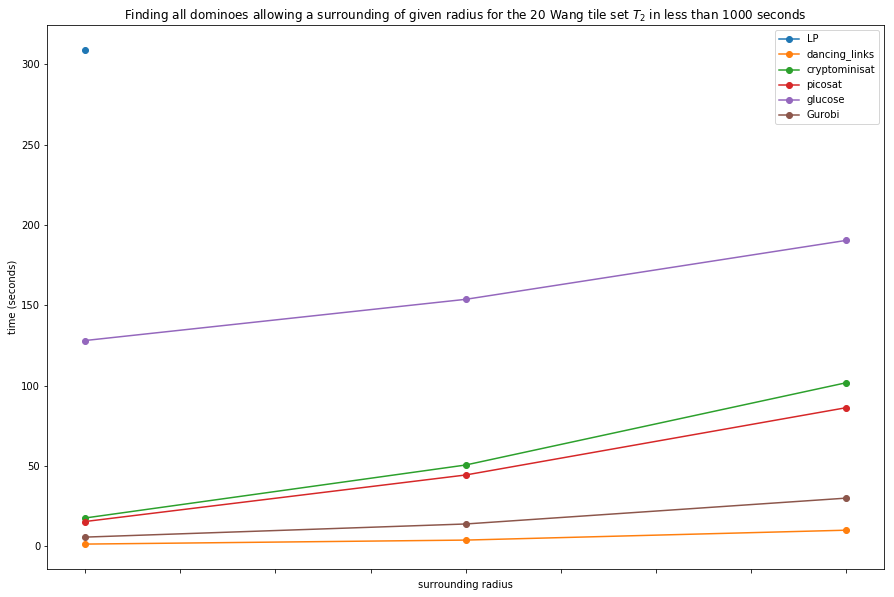In [ ]:


In :
df_dominoes_T3 = compare_solvers_for_dominoes(T3, solvers, radius_list=[1,2,3], timelimit=timelimit)
df_dominoes_T3

Testing solver dancing_links ...
-> Found (37, 36, 36) and (150, 135, 122) dominoes in the direction 1 and 2 resp for radius in [1, 2, 3]
-> time data: (2.529252052307129, 6.617175102233887, 14.96087908744812)
Testing solver cryptominisat ...
-> Found (37, 36, 36) and (150, 135, 122) dominoes in the direction 1 and 2 resp for radius in [1, 2, 3]
-> time data: (34.189846992492676, 96.47961091995239, 188.86419200897217)
Testing solver picosat ...
-> Found (37, 36, 36) and (150, 135, 122) dominoes in the direction 1 and 2 resp for radius in [1, 2, 3]
-> time data: (29.82133984565735, 86.3204460144043, 170.14684510231018)
Testing solver glucose ...
-> Found (37, 36, 36) and (150, 135, 122) dominoes in the direction 1 and 2 resp for radius in [1, 2, 3]
-> time data: (197.3815038204193, 242.88399982452393, 312.44361782073975)
Testing solver Gurobi ...
-> Found (37, 36, 36) and (150, 135, 122) dominoes in the direction 1 and 2 resp for radius in [1, 2, 3]
-> time data: (9.465282917022705, 24.315946102142334, 55.83455801010132)
Testing solver LP ...
-> Found (37,) and (150,) dominoes in the direction 1 and 2 resp for radius in [1, 2, 3]
-> time data: (659.5920040607452,)

Out:
LP dancing_links cryptominisat picosat glucose Gurobi
1 659.592004 2.529252 34.189847 29.821340 197.381504 9.465283
2 NaN 6.617175 96.479611 86.320446 242.884000 24.315946
3 NaN 14.960879 188.864192 170.146845 312.443618 55.834558
In :
ax = df_dominoes_T3.plot(figsize=(15,10), marker='o')
'for the 24 Wang tile set $T_3$ in less than {} seconds'.format(timelimit))